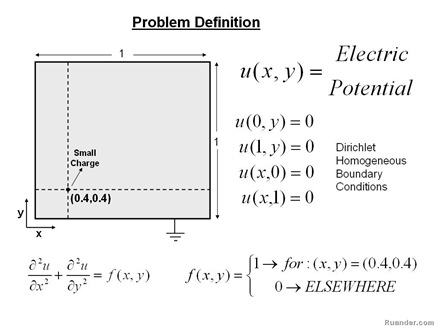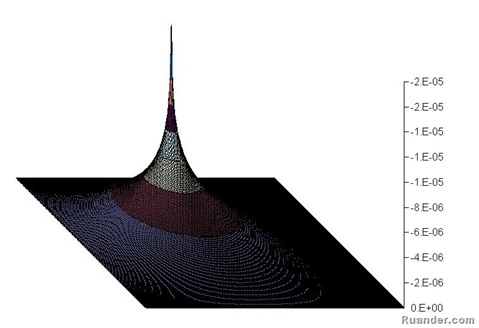## Pages

### Electric Potential Field around a charged particle

Any stationary charged particle creates an electric potential field around itself (electrostatics). Understanding the distribution of the electric potential filed is indispensable to understand the interactions that exist between charged particles and eventually electrical principles. The mathematical relationship that describes this process is an Elliptic Partial Differential Equation. Partial Differential equations are nowadays solved by numerical techniques. Elliptic PEDs in specific are solved by an iterative procedure.For example consider the example shown above. A small charged particle is located in a 2 dimensional square domain as shown. The edges of the domain are grounded, which means that the electric potential on the boundaries is forced to be zero. Without going into an extensive discussion on how to solve the problem it is just important to mention that: a) a consistent numerical scheme must be chosen, b) the domain needs to be dicretized in both directions with an appropriate step size, c) all the equations need to be written in a computational software (usually using index notation), including those for the boundary conditions. Once this is done, a solution is found iteratively by guessing an initial solution and iterate several times until one reaches a specified accuracy (residual). The solution for the example problem is shown below.